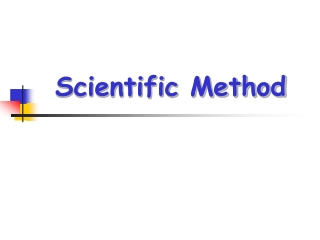DownloadDownload PresentationScientific Method

# Scientific Method

Download Presentation## Scientific Method

- - - - - - - - - - - - - - - - - - - - - - - - - - - E N D - - - - - - - - - - - - - - - - - - - - - - - - - - -
##### Presentation Transcript

1. Scientific Method

2. Steps in the Scientific Method • Observation • Hypothesis • Experiment • Data Collection • Conclusion • Retest

3. Observations • Gathered through your senses • A scientist notices something in their natural world

4. Observations • An exampleof an observation might be noticing that many salamanders near a pond have curved, not straight, tails

5. Hypothesis • A suggested solution to the problem. • Must be testable • Sometimes written as If…Then… statements • Predicts an outcome

6. Hypothesis • An example of a hypothesis might be that the salamanders have curved tails due to a pollutant in the moist soil where they live.

7. Experiment • A procedure to test the hypothesis.

8. Experiment Variable – factor in the experiment that is being tested

9. Experiment A good or “valid” experiment will only have ONE variable!

10. Controls and Variables

11. Scientific Experiments Follow Rules • An experimenter changes one factor andobserves or measureswhat happens.

12. The Control Variable • The experimenter makes a special effort to keep other factors constant so that they will not effect the outcome. • Those factors are called control variables.

13. What is the Purpose of a Control? • Controls are NOT being tested • Controls are used for COMPARISON

14. Other Variables • The factor that is changed is known as the independent variable. • The factor that is measured or observed is called the dependent variable.

15. Example of Controls & Variables • For example, suppose you want to figure out the fastest route to walk home from school. • You will try several different routes and time how long it takes you to get home by each one. • Since you are only interested in finding a route that is fastest for you, you will do the walking yourself.

16. What are the Variables in Your Experiment? • Varying the route is the independent variable • The time it takes is the dependent variable • Keeping the same walker throughout makes the walker a control variable.

17. One more thing… it is best to make several trials with each independent variable.

18. Valid Experiments

19. Remember: To be a Valid Experiment: • Two groups are required --- the control & experimental groups • There should be only onevariable

20. Data • Results of the experiment • May be quantitative (numbers) or qualitative

21. Data • Must be organized • Can be organized into charts, tables, or graphs

22. Conclusion • The answer to the hypothesis based on the data obtained from the experiment

23. Retest In order to verify the results, experiments must be retested.

24. Review

25. Solving a Problem 1)Identify a Problem 2) State Observations about the problem 3) Form a Hypothesis about the problem (if…then…) 4) Design an Experiment to test the hypothesis 5) Collect Data 6) Form a Conclusion 7) Retest